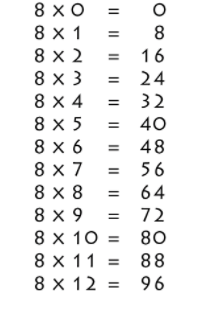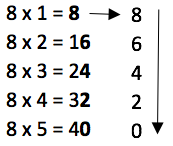# 8 Times Table- Learn Table Of 8 : Multiplication Table Of 8

Safalta expert Published by: Yashaswi More Updated Tue, 31 May 2022 12:17 AM IST

## Highlights

Check out how to learn the 8 Times table easily here at Safalta.com

The table of 8 shows what happens when you multiply 8 by other whole numbers. The ability to remember the 8 times table increases memory, which is a transferable talent that will aid pupils throughout their school careers and in life. Students who know the table of eights are more likely to be confident in their math skills. The number 8 has an interesting property in that when it is reversed, it becomes the sign of infinity (). This lesson will show you how to memorise an 8 times table, which is an eight-digit multiplication table for whole integers up to twenty. You may also read 4 Times Table- Learn Table Of 4

For example., Kelly reads 8 pages of a book each day. How many pages will she have read in 9 days? In this, the answer will be 8 x 9 = 72. Join Safalta School Online and prepare for Board Exams under the guidance of our expert faculty. Our online school aims to help students prepare for Board Exams by ensuring that students have conceptual clarity in all the subjects and are able to score their maximum in the exams.

## 8 times table Chart-## Multiplication Table of 8

• Learning the multiplication table of 8 helps you do mental math. This table comes in handy while solving problems in the real world.
• It saves time while doing long multiplication and division problems.
• The students will be able to recognize the multiplication sequence being followed with the successive multiples of 8.

Go through the 8 times table that is given below for quick calculations.

## 8 Times Table

8 Times Table up to 10

## Tips for 8 Times Table

1. 8 doesn’t have any rules that make its multiplication table of 8 easy to memorize, but there is a pattern for every five multiples of eight. 8, 16, 24, 32, 40, 48, 56, 64, 72, 80.

2. The last digit of these multiples always repeat, which means that students can remember these digits to help them with the 8 times table.

Another way to learn 8 Times Table is-You can notice in the above image that the digits which are in the unit's place are occurring in a sequence 0, 2, 4, 6, 8 from bottom to top and then recurring again. While in the tens place, the numbers are just increasing from top to bottom. We have obtained the first ten multiples of 8. Let us use the same pattern and create the 8 times table up to 20.

## Practise Questions

1. ### Example 1: Using the table of 8, evaluate 8 times 5 plus 3.

Solution:

This statement is expressed as 8 × 5 + 3

Now, from the 8 times table, we know that 8 times 5 is 40.

Therefore, 8 × 5 + 3 = 40 + 3 = 43

Hence, 8 times 5 plus 3 is 43.

2. ### Example 2: Check using the table of 8 whether 8 times 2 minus 6 is 10.

Solution:

First, we will write 8 times 2 minus 6 mathematically.

Using 8 times table, we have: 8 times 2 minus 6 = 8 × 2 - 6 = 16 - 6 = 10

Hence, 8 times 2 minus 6 is 10.

3. ### Example 3: Using 8 times table, find the value of 8 times 3 minus 10 plus 9.

Solution:

First, we will write 8 times 3 minus 10 plus 9 mathematically.

i.e. 8 times 3 minus 10 plus 9 = 8 × 3 - 10 + 9

Now from 8 times table, we have 24 - 10 + 9 = 24 - 1 = 23

Hence, 8 times 3 minus 10 plus 9 is 23.

## What is the multiplication table of 8?

8, 16, 24, 32, 40, 48, 56, 64, 72, 80.

## Does 100 come in the table of 8?

Forward counting by 8's: 0, 8, 16, 24, 32, 40, 48, 56, 64, 72, 80, 88, 96, 104, 112, 120, 128, 136, 144, 152, 160, 168, 176, 184, 192, 200,

## What are the multiples of 8?

The multiples of 8 are 8, 16, 24, 32, 40, 48, 56, 64, 72, 80… and so on. It is a sequence where the difference between each next number and the preceding number, i.e. two consecutive results, is 8.

## What is the importance of learning Table 2 to 20?

For making mathematical section easier, memorising table 2 to 20 is important.

## How can I learn tables easily?

Daily recite the table as mentioned in the article twice and thrice.

## Is 200 in the 8 times table?

Forward counting by 8's: 0, 8, 16, 24, 32, 40, 48, 56, 64, 72, 80, 88, 96, 104, 112, 120, 128, 136, 144, 152, 160, 168, 176, 184, 192, 200,